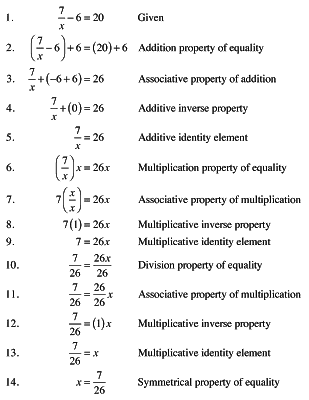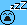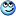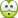# Missing out on intermediate algebraic steps

• B
This is going to sound very silly indeed, however I've been tutoring basic algebra and it's caused me think about a few little points that I'd never really even considered before.

With an equality ##a=b##, we might define a function ##f## representing a certain operation we could perform on both sides of the equation, like for example ##f(x) = 3x##. Since, if ##a=b## then ##f(a) = f(b)##, this then formalises the various properties of equality because we can apply ##f## to both sides and obtain ##3a=3b##, etc.

If our equation was ##\frac{3}{(x+2)} = 6##, "formally" we'd use ##a=b \implies ac=bc## to do the following two steps $$\frac{3}{(x+2)}(x+2) = 6(x+2)$$ $$3 = 6(x+2)$$ Normally, I think anyone would have just skipped to the second line, though I wondered if the above example counts as two different steps - the first being applying the operation, and the second simplifying? Do these count as distinct steps? I'm aware this essentially changes nothing, though just found it a little curious. If we're working with matrices or groups, it's less common to see the intermediate step skipped, i.e. $$ab=c$$ $$a^{-1}ab = a^{-1}c \quad \text{(apply inverse operation)}$$ $$eb= a^{-1}c \quad \text{(simplify)}$$ $$b = a^{-1}c \quad \text{(simplify)}$$Thanks, and please do advise if I'm rambling!

Last edited by a moderator:

It's good you think about these kind of things but whether this is classified as one step or two steps is a non-mathematical discussion. It depends on the expertise of the person that uses it.

I wouldn't write a single thing as this equation is so simple to me it doesn't need any calculation, but if you are tutoring something you might want to write out all steps, even the intermediate simple ones.

Also, in general you have to be careful when applying a function ##f## to both sides that is non-injective. I.e. for example

##x = y \implies x^2 = y^2## but not conversely, so in the end you have to check that you didn't introduce non-solutions to the problem you started with.

•etotheipi
It's good you think about these kind of things but whether this is classified as one step or two steps is a non-mathematical discussion. It depends on the expertise of the person that uses it.

I wouldn't write a single thing as this equation is so simple to me it doesn't need any calculation, but if you are tutoring something you might want to write out all steps, even the intermediate simple ones.

Yeah, I see what you mean. If I write down ##\frac{1}{3}x = 5## and say multiply both sides by 3, it's perfectly reasonable to go straight to ##x=15##, since both sides have been multiplied by 3. But to get there, you have to at least imagine the step ##3(\frac{1}{3}x) = 3(5)## in order to perform the computation.

In a sense, it's still there but it's just been skipped over. As you say, it's not really a mathematical discussion. It's just a little peculiar to think about.

Homework Helper
Gold Member
2022 Award
You can think of every step as the application of an axiom. And in some formal mathematics courses that's what you have to do. At every step you declare what axiom you are using. For example:

$$ab=c$$ $$a^{-1}ab = a^{-1}c \quad \text{(apply inverse operation)}$$ $$eb= a^{-1}c \quad \text{(simplify)}$$ $$b = a^{-1}c \quad \text{(simplify)}$$

You could also indicate where you are using the associative law. This gets pretty tedious for most people though.

•etotheipi
You can think of every step as the application of an axiom. And in some formal mathematics courses that's what you have to do. At every step you declare what axiom you are using. For example: ...

You could also indicate where you are using the associative law. This gets pretty tedious for most people though.

I think this is a good way to define a step. And it also demonstrates why skipping steps is so common (and in fact, important, for the sake of global ink supplies). If you wanted to solve ##3(x+2) = 7##, then you'd essentially be doing $$3x+6 = 7 \quad \text{(distribute)}$$ $$3x + 6 - 6 = 7 - 6 \quad \text{(subtract)}$$ $$3x = 1 \quad \text{(cancel inverses)}$$ $$\frac{1}{3}(3x) = \frac{1}{3}(1) \quad \text{(multiply)}$$ $$x = \frac{1}{3} \quad \text{(cancel inverses)}$$ When of course, one line often suffices. Transposition of a term from one side to the other is really two steps, disguised as one, etc.

Actually, seems I was skimping. I thought this was quite funny, courtesy of Cary MillsapHomework Helper
Gold Member
2022 Award•etotheipiThe best way is to write a Python script which checks infinitely many numbers. Then we don't even need to worry about the silly axioms.

Mentor
If our equation was ##\frac{3}{(x+2)} = 6##, "formally" we'd use ##a=b \implies ac=bc## to do the following two steps $$\frac{3}{(x+2)}(x+2) = 6(x+2)$$ $$3 = 6(x+2)$$
Something not mentioned in the thread is the difference between multiplying both sides of an equation by a constant versus multiplying by a variable quantity.
As an example, consider this equation:
$$\frac{x^2 - 1}{x - 1} = 2$$
If we apply the same logic as I quoted above, we get ##x^2 - 1 = 2x - 2##. A bit more work yields the equation ##x^2 -2x + 1 = 0##, or ##(x - 1)^2 = 0##, from which we conclude that x = 1.
However, if we replace x by 1 in the original equation, we end up with ##\frac 0 0 = 2##, which is nonsense.
Regarding the equation of post #1, if you're working with rational equations, it's always a good idea to explicitly state what values of the variable aren't allowed.

•etotheipi
Homework Helper
2022 Award
If we're working with matrices or groups, it's less common to see the intermediate step skipped, i.e.
This is doubtless subsumed in the previous discussion but particularly the existence of the inverse needs some consideration in these circumstances. Failure to do so is not so good

•etotheipi

I think it's easiest to explain applying the two steps separately. It's like if we have the equation ##e^{3x} = 5##. When we say "take logs of both sides", it refers to the step just pertaining to applying the function:

##\ln(e^{3x}) = \ln(5)##

and then in a further step we can use the properties of inverse functions

##3x = \ln(5)##

The same goes for addition/multiplication etc, "adding to both sides" should only refer to the intermediate step (i.e. applying the function ##f(x) = x + c##), before simplifying. Though in practice, one lumps both steps under the umbrella term of "adding to both sides".

Anyways, I think we've discussed all there is to it, so I won't burden anyone by dragging this out any longer. Thank you!

Last edited by a moderator:
Something not mentioned in the thread is the difference between multiplying both sides of an equation by a constant versus multiplying by a variable quantity.

Even "adding to both sides" is not safe. Consider the equation: ## \frac{1}{x-2} + x = \frac{1}{x-2} + 2##.

When ##x = 2## the expression ##\frac{1}{x-2}## is not "a quantity".

This thread focuses on procedures for manipulating symbols. Such procedures are only justified if they can be interpreted as coherent mathematical statements. Whether students should be taught a coherent interpretation for "doing the same things to both sides of an equation" before or after they learn the concept is an interesting question.

The fundamental idea is that equations have solution sets. "Doing the same thing to both sides" may or may not produce a different equation with the same solution set. Justification for the symbolic manipulations involved in "doing the same thing to both sides" must be arguments that the new equation has the same solution set as the original, or that it has the same solution set provided certain conditions or exception are met. It's tedious to write out such arguments using complete sentences. Hence we settle for some compromise to being coherent - such as "check your answers in the original equation". Checking answers in the original equation is a procedure, not a explanation.

•SammyS
This thread focuses on procedures for manipulating symbols. Such procedures are only justified if they can be interpreted as coherent mathematical statements. Whether students should be taught a coherent interpretation for "doing the same things to both sides of an equation" before or after they learn the concept is an interesting question.

Do you think the notion of "doing the same thing to both sides" is a helpful one? It appears one can get by just fine without it just by using the axioms $$a + b = c \quad \text{(given)}$$ $$a + b + (-c) = c + (-c) \quad \text{(substitution axiom)}$$ $$a + b - c = 0 \quad \text{(additive inverse)}$$

Homework Helper
Gold Member
Keeping with "Intermediate Algebra" and the originally posted example, easily many students can and will jump a step or two in their heads. Some people need a little practice; and some may need more practice. Usually only a concern for beginners, unfamiliar with simple arithmetic Algebra.

If you feel you must write more individual steps, then do that.

•etotheipi
Keeping with "Intermediate Algebra" and the originally posted example, easily many students can and will jump a step or two in their heads. Some people need a little practice; and some may need more practice. Usually only a concern for beginners, unfamiliar with simple arithmetic Algebra.

If you feel you must write more individual steps, then do that.

Before I didn't really need to think about it, since I suppose I'd just gotten "used" to all of the manipulations so could generally do the rearrangements mentally and write down an answer.

However when I then try to explain to students who are relatively new to the subject, I find myself struggling to paint a coherent fundamental picture. Lots of it is just tiny details, but it just bothers me.

To give another really dull example, one might write ##4x + 2 = 4## and then ##4x = 2## on the next line. We might say that we've subtracted 2 from both sides, but in reality subtracting 2 from both sides only gets us to ##4x + 2 -2 = 2-2##. We need another step to complete the simplification.

I don't know why I'm worrying so much about this, but it just peeves me a little bitDo you think the notion of "doing the same thing to both sides" is a helpful one?
It is helpful as a general guide to doing symbolic manipulations. It's like such guides as "combine like terms" or the "FOIL method". These are guides for doing procedures, not guides for making mathematical deductions. They help make deductions only insofar as the procedures are reliable.

It appears one can get by just fine without it just by using the axioms $$a + b = c \quad \text{(given)}$$ $$a + b + (-c) = c + (-c) \quad \text{(substitution axiom)}$$ $$a + b - c = 0 \quad \text{(additive inverse)}$$

In one sense, yes, in elementary algebra. But you haven't made a specific mathematical claim to define what "do just fine" and "using the axioms" means. It's one thing to explain to students a procedure and a different thing to explain to them what arguments justify the procedure.

One consideration is that many students won't pay attention to arguments that justify the procedure and will only focus on doing the problems with symbolic manipulations. So, from a the viewpoint of getting them ready for standardized tests, teaching the manipulations is the crucial material. You are attempting to be precise about critera for the steps in a symbolic procedure written in the step-reason format. The step-reason format isn't, by itself, any sort of explanation. (Try stating what a step-reason entry claims using complete sentences.) It's not possible to define any sort of logically consistent criteria to judge the correctness of writing in the step-reason format without translating that format into coherent mathematical statements. In the mind of most students the criteria for the correctness of the step-property format will be based on recognizing patterns that "the teacher wants us to use", not on being able interpret the format.

For example:

##x = 2 - 2##
##x = 0## reason: arithmetic

versus

##x = 2 - 2##
##x = 2 + (-2) ## reason: definition of subtraction
##x = 0 ## reason: definition of the additive inverse of 2

•etotheipi
The step-reason format isn't, by itself, any sort of explanation. (Try stating what a step-reason entry claims using complete sentences.) It's not possible to define any sort of logically consistent criteria to judge the correctness of writing in the step-reason format without translating that format into coherent mathematical statements.

So if I start off with ##x + 2 = 2##, and my step-reason format goes a bit like $$x+ 2 =2 \quad \text{(given)}$$ $$x + 2 + (-2) = 2 + (-2) \quad \text{(addition property)}$$ $$x + 0 = 0 \quad \text{(additive inverse)}$$ $$x = 0 \quad \text{(additive identity)}$$ Couldn't I rewrite that in terms of three statements
1. Since ##x + 2 + (-2) = x + 2 + (-2)## due to reflexivity and ##x + 2 = 2##, as given, then ##x + 2 + (-2) = 2 + (-2)##
2. ##2## and ##-2## are additive inverses so ##2 + (-2)## evaluates to the identity, ##0##.
3. ##0## is the additive identity so ##x + 0 = x##
Is this what you mean?

Homework Helper
Gold Member
Regard to posts #16 and #17, one should quickly recognize the definitions and the properties and be able to use them, but should not need to break every single expression into every single possible step most of the time.

•etotheipi
Regard to posts #16 and #17, one should quickly recognize the definitions and the properties and be able to use them, but should not need to break every single expression into every single possible step most of the time.

Yes, I think this is the moral of the story, and it's fine to just keep the rest at the back of one's mind. Though I think I'm more comfortable reserving the term "add ##\text{X}## to both sides" for the step in which we append ##+53## to both sides, and haven't yet simplified. In that sense, "add ##\text{X}## to both sides" really just translates to "apply the addition property of equality with ##f(x) = x + \text{X}##".

Really, we should be saying "add 3 to both sides and simplify" when going from ##x-3 = 1## to ##x=4##.

Not that any of this really matters a great deal!

Homework Helper
Gold Member
Yes, I think this is the moral of the story, and it's fine to just keep the rest at the back of one's mind. Though I think I'm more comfortable reserving the term "add ##\text{X}## to both sides" for the step in which we append ##+53## to both sides, and haven't yet simplified. In that sense, "add ##\text{X}## to both sides" really just translates to "apply the addition property of equality with ##f(x) = x + \text{X}##".

Really, we should be saying "add 3 to both sides and simplify" when going from ##x-3 = 1## to ##x=4##.

Not that any of this really matters a great deal!
But any of this DOES matter. Some of what we do becomes automatic. We just learn to recognize what is displayed on paper/ on board in front of us, almost as if it was some blend of simple mechanical construct and ingrained language behavior. We get that way through both study and practice.

•etotheipi
So if I start off with ##x + 2 = 2##, and my step-reason format goes a bit like $$x+ 2 =2 \quad \text{(given)}$$ $$x + 2 + (-2) = 2 + (-2) \quad \text{(addition property)}$$ $$x + 0 = 0 \quad \text{(additive inverse)}$$ $$x = 0 \quad \text{(additive identity)}$$ Couldn't I rewrite that in terms of three statements
1. Since ##x + 2 + (-2) = x + 2 + (-2)## due to reflexivity and ##x + 2 = 2##, as given, then ##x + 2 + (-2) = 2 + (-2)##
2. ##2## and ##-2## are additive inverses so ##2 + (-2)## evaluates to the identity, ##0##.
3. ##0## is the additive identity so ##x + 0 = x##
Is this what you mean?

You have the general idea, but your explanation using complete sentences doesn't explain what "addition property" states. Addition property refers to a theorem. What is the statement of that theorem as a complete sentence?

I think the argument should be written in way that makes it clear whether the transition between steps is and if-then argument versus and if-and-only-if argument.

For example:
Given ##x = 2 + (-2)##
##x = 0## reason: definition of the additive inverse of 2

can be interpreted as the claim " ##x = 2 + ( -2) ## if and only if ##x = 0##".

Technically that claim cannot be asserted a single step. The justification, given ##x = 2 + (-2)##, is that ##2 + (-2) = 0## by definition of the additive inverse of 2. Then we use the transitive property of "=" to argue that "##x = 2 + (-2)## and ## 2 + (-2) = 0##" implies ##x = 0##.

What I think you mean by "addition property" is the theorem: If ##a##, ##b##, and ##c## are numbers then: ##a = b## if and only if ##a + c = b + c##.

The stipulation that the symbols represent numbers is important. Symbolic expressions such as ##\frac{1}{x-2}## do not always represent numbers (e.g. when x = 2).

•etotheipi
Homework Helper
Gold Member
Stephen Tashi,

I wonder from what point of view do you handle the discussion in your post #21?
(Guessing, Algebra at an advanced level)

The fact that we have these very solidly reliable statements of number properties is reassuring. Those properties gives Arithmetic and Algebra practitioners power. We DO use them, but when we transform expressions or solve equations or inequalities, we can and often do jump steps, and usually not resort to writing each step exactly according to these properties - but our steps DO very much rely on our understanding and use of these properties (I'm trying my best to say this right).

Last edited:
•etotheipi
The fact that we have these very solidly reliable statements of number properties is reassuring. Those properties gives Arithmetic and Algebra practitioners power. We DO use them, but when we transform expressions or solve equations or inequalities, we can and often do jump steps, and usually not resort to writing each step exactly according to these properties - but our steps DO very much rely on our understanding and use of these properties (I'm trying my best to say this right).

I agree that teaching procedures of solving equations in introductory algebra need not necessarily involve teaching the interpretation of such procedures. Writing solutions in the step-reason form is a compromise between a mechanical procedure and a coherent mathematical argument presented using complete sentences. However, the step-reason format is not a mathematical statement of what is being claimed or an explicit statement of why the claims are correct. Few students in introductory algebra are capable of understanding the distinction between a coherent mathematical argument and symbolic manipulations. I'm aware that very few students and, probably, very few teachers, can put into complete sentences the arguments that interpret the steps in algebraic manipulations.

I'm not advocating that every demonstration of solving an equation should be in the step-reason form or in a more coherent form. It is true that much of the "power" of mathematics is the ability to do manipulations without thinking about what they mean. However, the standard on a math forum should be logical clarity. So when the discussion gets into questions of why or why not some procedures are correct, it needs to get to the bottom of things.

$$\frac{x^2 - 1}{x - 1} = 2$$